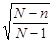Wald interval (aka one proportion z-interval):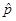+ z*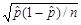Score interval (aka Wilson) inverts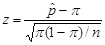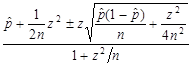The midpoint of the Score interval can be rewritten as:(n /(n + z2)) + .5(z2/(n+z2)), a weighted average ofand 0.50.

Adjusted Wald interval: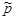+ z*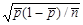where=  (X+.5z*2)/(n+z*2), ñ= n+z*2

For 95% confidence this is approximately:= (X+2)/(n+4) and ñ = n+4 which adds two successes and two failures to the sample (“plus four” method)

Finite Population Correction Factor (N = population size)+ z*